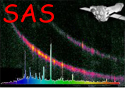XMM-Newton Science Analysis System

rgssources (rgssources-6.3.3) [xmmsas_20230412_1735-21.0.0]

## CONFUSION values.

It is intended to flag in the RGS pipeline sources which are confused with the prime source, so as to allow the end user to assess whether these have a deleterious effect on the spectra of the prime source. For this purpose rgssources calculates, for each source, a value which is stored in a column called CONFUSION. This value is calculated from the following formula:Hereis the confusion value,is the source brightness (ie value of the RATE column) andandare respectively the dispersion and cross-dispersion angles in arcseconds. The constants,,,,andwill eventually be stored in CCF MiscData, but at present are `hard-wired' into the task code, with values as follows:XMM-Newton SOC -- 2023-04-16